# Fraction calculator

The calculator performs basic and advanced operations with fractions, expressions with fractions combined with integers, decimals, and mixed numbers. It also shows detailed step-by-step information about the fraction calculation procedure. Solve problems with two, three, or more fractions and numbers in one expression.

## Result:

### (5/6) : (1/3) = 5/2 = 2 1/2 = 2.5

Spelled result in words is five halfs (or two and one half).

### How do you solve fractions step by step?

1. Divide: 5/6 : 1/3 = 5/6 · 3/1 = 5 · 3/6 · 1 = 15/6 = 3 · 5 /3 · 2 = 5/2
Dividing two fractions is the same as multiplying the first fraction by the reciprocal value of the second fraction. The first sub-step is to find the reciprocal (reverse the numerator and denominator, reciprocal of 1/3 is 3/1) of the second fraction. Next, multiply the two numerators. Then, multiply the two denominators. In the next intermediate step, , cancel by a common factor of 3 gives 5/2.
In words - five sixths divided by one third = five halfs.

#### Rules for expressions with fractions:

Fractions - use the slash “/” between the numerator and denominator, i.e., for five-hundredths, enter 5/100. If you are using mixed numbers, be sure to leave a single space between the whole and fraction part.
The slash separates the numerator (number above a fraction line) and denominator (number below).

Mixed numerals (mixed fractions or mixed numbers) write as non-zero integer separated by one space and fraction i.e., 1 2/3 (having the same sign). An example of a negative mixed fraction: -5 1/2.
Because slash is both signs for fraction line and division, we recommended use colon (:) as the operator of division fractions i.e., 1/2 : 3.

Decimals (decimal numbers) enter with a decimal point . and they are automatically converted to fractions - i.e. 1.45.

The colon : and slash / is the symbol of division. Can be used to divide mixed numbers 1 2/3 : 4 3/8 or can be used for write complex fractions i.e. 1/2 : 1/3.
An asterisk * or × is the symbol for multiplication.
Plus + is addition, minus sign - is subtraction and ()[] is mathematical parentheses.
The exponentiation/power symbol is ^ - for example: (7/8-4/5)^2 = (7/8-4/5)2

#### Examples:

adding fractions: 2/4 + 3/4
subtracting fractions: 2/3 - 1/2
multiplying fractions: 7/8 * 3/9
dividing Fractions: 1/2 : 3/4
exponentiation of fraction: 3/5^3
fractional exponents: 16 ^ 1/2
adding fractions and mixed numbers: 8/5 + 6 2/7
dividing integer and fraction: 5 ÷ 1/2
complex fractions: 5/8 : 2 2/3
decimal to fraction: 0.625
Fraction to Decimal: 1/4
Fraction to Percent: 1/8 %
comparing fractions: 1/4 2/3
multiplying a fraction by a whole number: 6 * 3/4
square root of a fraction: sqrt(1/16)
reducing or simplifying the fraction (simplification) - dividing the numerator and denominator of a fraction by the same non-zero number - equivalent fraction: 4/22
expression with brackets: 1/3 * (1/2 - 3 3/8)
compound fraction: 3/4 of 5/7
fractions multiple: 2/3 of 3/5
divide to find the quotient: 3/5 ÷ 2/3

The calculator follows well-known rules for order of operations. The most common mnemonics for remembering this order of operations are:
PEMDAS - Parentheses, Exponents, Multiplication, Division, Addition, Subtraction.
BEDMAS - Brackets, Exponents, Division, Multiplication, Addition, Subtraction
BODMAS - Brackets, Of or Order, Division, Multiplication, Addition, Subtraction.
GEMDAS - Grouping Symbols - brackets (){}, Exponents, Multiplication, Division, Addition, Subtraction.
Be careful, always do multiplication and division before addition and subtraction. Some operators (+ and -) and (* and /) has the same priority and then must evaluate from left to right.

## Fractions in word problems:

• The thirdThe one-third rod is blue, one-half of the rod is red, the rest of the rod is white and measures 8 cm. How long is the whole rod?
• Gear wheelsTwo gear wheels, which fit together, have the number of teeth z1=58 and z2=149. Calculate the speed of the first wheel, if the second wheel rotates 1232 revolutions per minute.
• Drinking waterA man drinks a keg of water in 40 days, and a woman drinks in 62 days. How many days do they consume a keg together?
• Women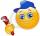In a company of 1050 employees are 2/3 women. How many women work in the company?
• Lemonade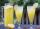How many 1/4 cup servings are in 2 and 1/3 cups of lemonade?
• 6/24 change6/24 change to a fraction in lowest terms
• JordanJordan wants to bring in cookie cakes to share with his 23 classmates for his birthday. His mom is willing to buy no more than 6 cookie cakes and Jordan must share the cakes equally between his classmates (and himself). Answer the questions to help Jordan
• The carpentersThe carpenters cut the beam. Half of the cut pieces were 1 meter long, a quarter of the pieces were two meters long and the remaining 8 meters of the beam were cut into two equal parts. - What was the length of the original beam? - What part of the origin
• Dana helped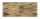Dana helped her dad build a sandbox for her younger sister. The sandbox is shaped like a rectangular prism that is 4 1/2 feet long and 4 feet wide. Dana used bags of sand to fill the sandbox 1/2 of a foot deep. Each bag contained 1/2 of a cubic foot of sa
• ŽSRCalculate fixed annual personnel costs of operating monorail line 118 km long if every 5 km is a station, which serves three people - one dispatcher and two switchmen in 4-shift operation. Consider the average salary of the employee 885 €.
• Camp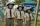In the camp are children. 1/2 went on a trip, 1/4 went to bathe, and 38 children remained in the room. How many children are in the camp?
• Metallurgical enterprise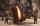Metallurgical company daily produces 318 tons of cast iron. How many tons of coke it burns to melt cast iron when 10 tons of cast consumes 6 tons of coke?
• Collection of stampsJano, Rado, and Fero have created a collection of stamps in a ratio of 5: 6: 9. Two of them had 429 stamps together. How many stamps did their shared collection have?